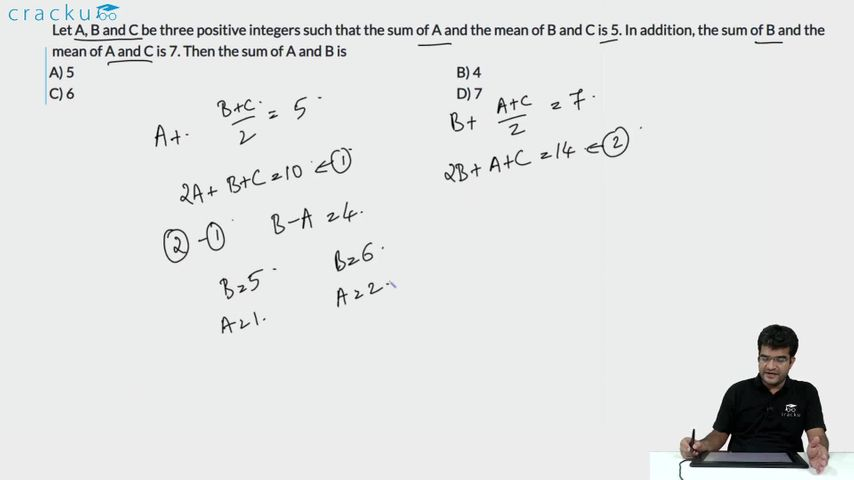Question 76

# Let A, B and C be three positive integers such that the sum of A and the mean of B and C is 5. In addition, the sum of B and the mean of A and C is 7. Then the sum of A and B is

Solution

Given

A+(B+C)/2=5 => 2A+B+C=10....(i)

(A+C)/2 +B=7 => A+2B+C=14.....(ii)

(i)-(ii)=> B-A=4 => B=4+A.

Given, A, B, C are positive integers

If A=1=>B=5 => C=3

If A=2=>B=6 => C=0 but this is invalid as C is positive.

Similarly if A>2 C will be negative and cases are not valid.

Hence, A+B=6.

### View Video Solution• All Quant CAT Formulas and shortcuts PDF
• 30+ CAT previous papers with solutions PDF